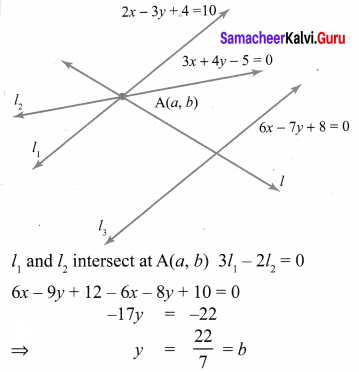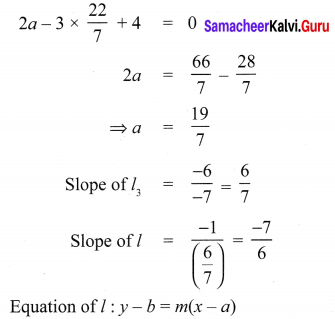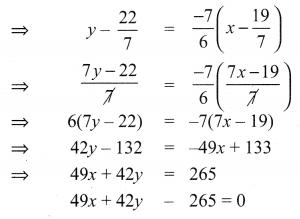You can Download Samacheer Kalvi 10th Maths Book Solutions Guide Pdf, Tamilnadu State Board help you to revise the complete Syllabus and score more marks in your examinations.

## Tamilnadu Samacheer Kalvi 10th Maths Solutions Chapter 5 Coordinate Geometry Unit Exercise 5

Question 1.
PQRS is a rectangle formed by joining the points P(-1, -1), Q(-1, 4) ,R(5, 4) and S(5, -1). A, B, C and D are the mid-points of PQ, QR, RS and SP respectively. Is the quadrilateral ABCD a square, a rectangle or a rhombus? Justify your answer.
Solution:
A, B, C and D are mid points of PQ, QR, RS & SP respectively.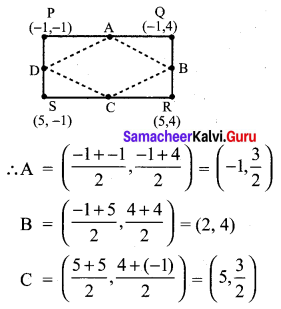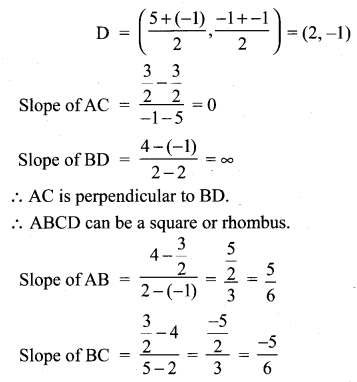∴ AB and BC are not perpendicular
⇒ ABCD is rhombus as diagonals are perpendicular and sides are not perpendicular.Question 2.
The area of a triangle is 5 sq.units. Two of its vertices are (2, 1) and (3, -2). The third Vertex is (x, y) where y = x + 3 . Find the coordinates of the third vertex.
Solution:
Area of triangle formed by points (x1, y1),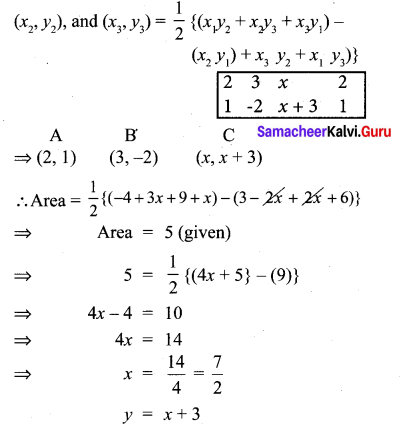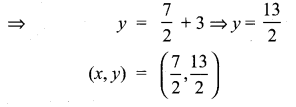Question 3.
Find the area of a triangle formed by the lines 3x + y – 2 = 0, 5x + 2y – 3 = 0 and 2x – y – 3 = 0
Solution: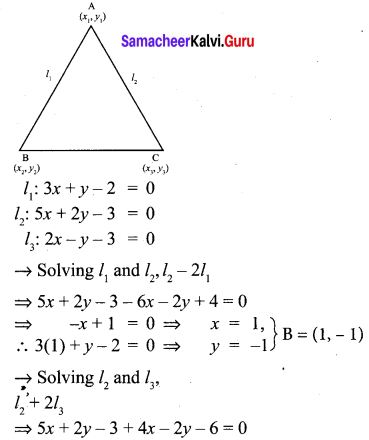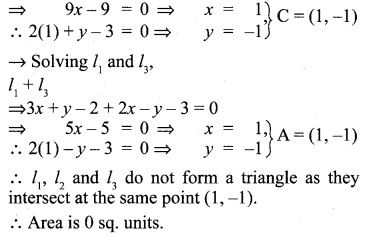Question 4.
If vertices of a quadrilateral are at A(-5, 7), B(-4, k) , C(-1, -6) and D(4, 5) and its area is
72 sq.units. Find the value of k.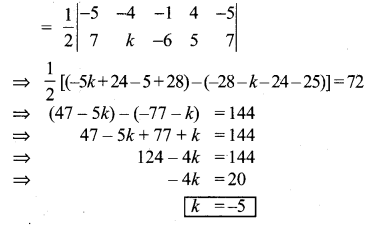Question 5.
Without using distance formula, show that the points (-2, -1) , (4, 0) , (3, 3) and (-3, 2) are vertices of a parallelogram.
Solution: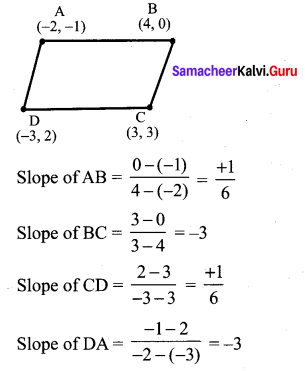Slope of AB = Slope of CD
Slope of BC = Slope of DA
Hence ABCD forms a parallelogram.

Question 6.
Find the equations of the lines, whose sum and product of intercepts are 1 and -6 respectively.
Let the intercepts be x1, y1 respectively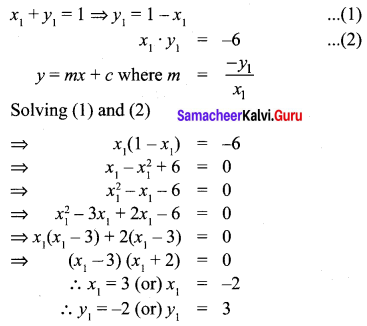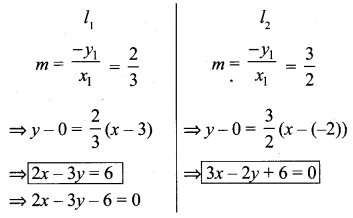Question 7.
The owner of a milk store finds that, he can sell 980 litres of milk each week at ₹ 14/litre and 1220 litres of milk each week at ₹ 16 litre. Assuming a linear relationship
between selling price and demand, how many litres could he sell weekly at ₹ 17/litre?
Solution: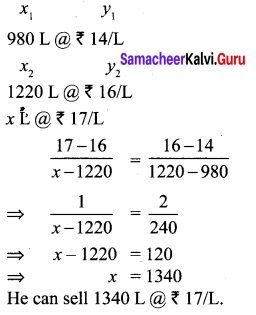Question 8.
Find the image of the point (3, 8) with respect to the line x + 3y = 7 assuming the line to be a plane mirror.
Solution: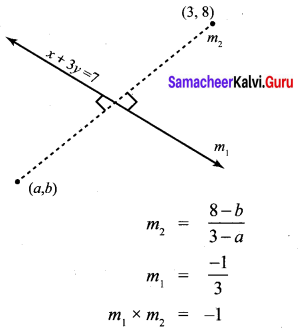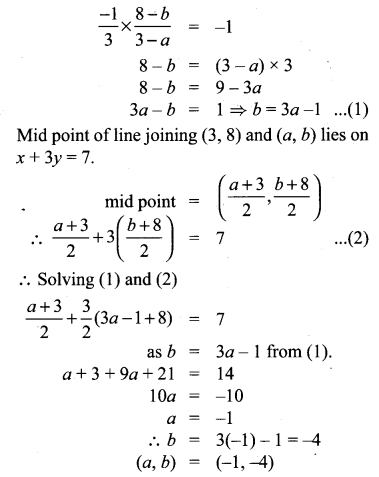Question 9.
Find the equation of a line passing through the point of intersection of the lines 4x + 7y – 3 = 0 and 2x – 3y + 1 = 0 that has equal intercepts on the axes.
Solution:
4x + 7y – 3 = 0
2x – 3y + 1 = 0
4x + 7y – 3 – 2(2x – 3y + 1) = 0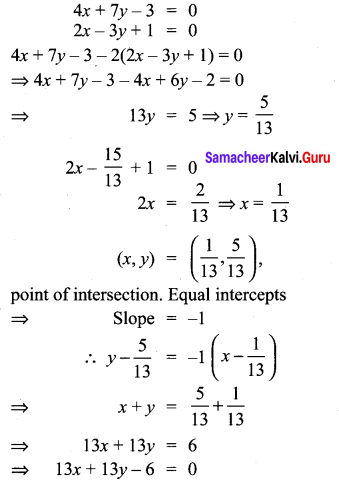Question 10.
A person standing at a junction (crossing) of two straight paths represented by the equations 2x – 3y + 4 = 0 and 3x + 4y – 5 = 0 seek to reach the path whose equation is 6x – 7y + 8 = 0 in the least time. Find the equation of the path that he should follow.
Solution: